# Alcohols

Alcohols are organic compounds containing a $-OH$ functional group. Two methods of producing ethanol are shown below:

### Hydration of Ethene

This is where ethanol is made industrially by the catalytic conversion of ethene, $C_{2}H_{4}$ using steam in the presence of a phosphoric acid catalyst, ($H_{3}PO_{4}$). This reaction is carried out at high temperature and pressure.

$$H_{2}C{=}CH_{2} + H_{2}O \rightarrow CH_{3}CH_{2}OHCH_{3}$$

This is a reversible reaction, so the conversion of ethene is incomplete with any unreacted gases recycled and passed through the reactor again.

### Fermentation of Sugars

This is where carbohydrates are converted into ethanol and carbon dioxide. The conditions for this are:

• Low temperatures around 37° where the yeast performs the best. Higher than this temperature, the yeast enzyme denatures and below this temperature the reaction is slow.
• Anaerobic conditions.

$$C_{6}H_{12}O_{6(aq)} + \rightarrow 2CH_{3}CH_{2}OHCH_{3(aq)} + 2CO_{2(g)}$$

## Properties of Alcohols

The physical properties of alcohols are influenced by their ability to form hydrogen bonds between $-OH$ groups of neighbouring molecules.

### Volatility and boiling points

Hydrogen bonds are the strongest type of intermolecular forces. The relatively high melting and boiling points of alcohols are due to the presence of hydrogen bonds between the alcohol molecules.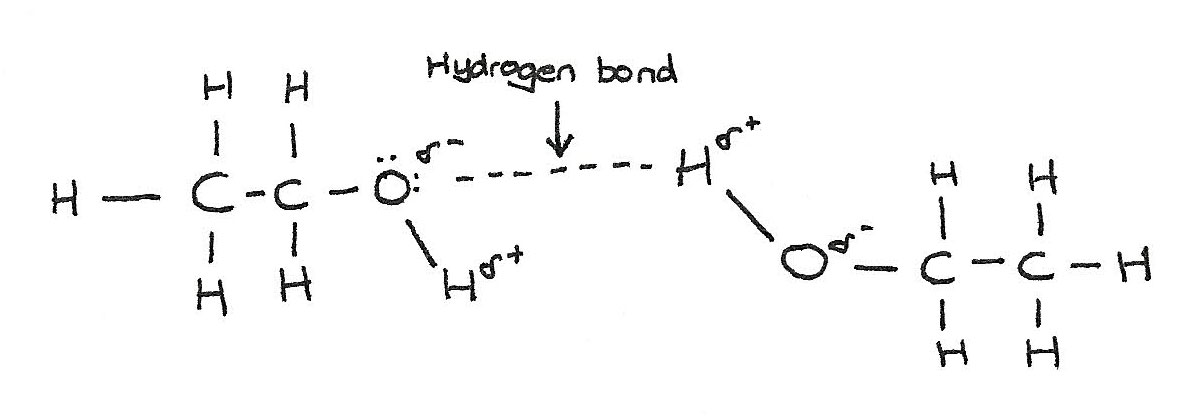The presence of hydrogen bonds result in them having a lower volatility than alkanes of similar mass. The boiling point of alcohols increase with longer chains.

### Solubility

Alcohols dissolve in water because hydrogen bonds form between the polar $-OH$ group of the alcohol and the water molecules.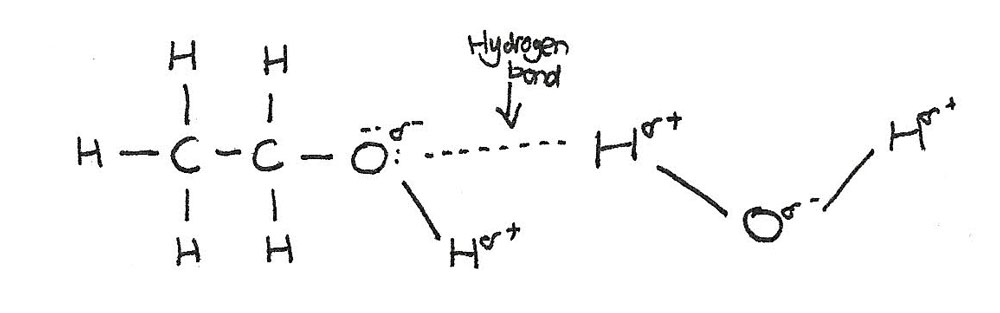Solubility decreases as the chain length increases. This is because a large part of the molecule is made up from a non-polar hydrocarbon chain. This chain is not able to form hydrogen bonds with the water molecules.

## Classification of Alcohols

Alcohols can be classed as primary, secondary or tertiary alcohols depending on the number of allyl groups attached to the carbon carrying the alcohol group.

### Primary alcohols

The $-OH$ atom is attached to a carbon with no bonded allyl groups.### Secondary alcohols

The $-OH$ group is attached to a carbon atom which is bonded to two alkyl groups.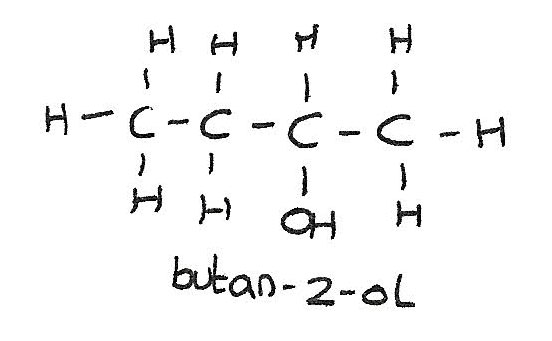## Tertiary alcohols

The $-OH$ group is bonded to a carbon atom which is bonded to three alkyl groups.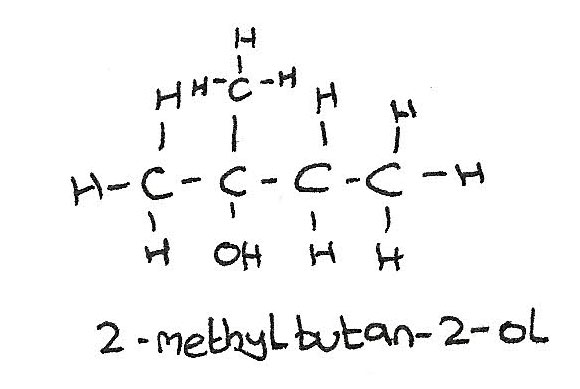## Combustion of alcohols

In a plentiful supply of oxygen, alcohols completely burn to form carbon dioxide and water.
$$C_{2}H_{6}O + 3O_{2} \rightarrow 2CO_{2} + 3H_{2}O$$

## Oxidation of alcohols

Primary and secondary alcohols can be oxidised using an oxidising agent. A suitable oxidising agent is a solution containing acidified dichromate ions, $H^+$ / $Cr_{2}O_{7}^{\kern{8px}2-}$. This oxidising mixture can be made from potassium dichromate, $K_{2}Cr_{2}O_{7}$ and sulphuric acid, $H_{2}SO_{4}$.

During the reaction, the acidified potassium dichromate changes colour from orange to green.

### Primary alcohols

Upon gentle heating with potassium dichromate, a primary alcohol can be oxidised to produce an aldehyde.
$$C_{3}H_{7}OH + [O] \rightarrow C_{2}H_{5}CHO + H_{2}O$$

On stronger heating under reflux and an excess of acidified potassium dichromate, alcohol is completely oxidised to form a carboxylic acid.
$$C_{3}H_{7}OH + 2[O] \rightarrow C_{2}H_{5}COOH + H_{2}O$$

When preparing aldehydes, it must be distilled off as it to formed. This prevents the further oxidisation to a carboxylic acid.

### Reflux

This is the continual boiling and condensing of a reaction mixture to ensure that the reaction takes place without the contents of the flask boiling dry.

### Secondary alcohols

Secondary alcohols are oxidised by acidified dichromate ions to produce ketones. Ketones cannot be oxidised further.

$$CH_{3}CH_{2}CH_{3} + [O] \rightarrow CH_{3}COCH_{3} + H_{2}O$$

### Tertiary alcohols

Tertiary alcohols are resistant to oxidation. The oxidising agent remains orange.

## Esterification

An ester is formed when an alcohol is warmed with a carboxylic acid in the presence of an acid catalyst. In the reaction below, methanol reacts with propanoic acid to produce the ester methyl propanoate and water.

$$CH_{3}CH_{2}COOH + HOCH_{3} \rightarrow CH_{3}CH_{2}COOCH_{3} + H_{2}O$$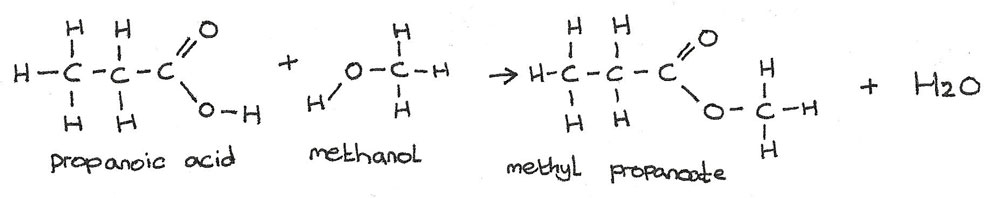## Dehydration of Alcohols

An alcohol can be dehydrated to form an alkene in the presence of an acid catalyst. This is an example of an elimination reaction. The alcohol is heated under reflux for 40 minutes.

$$C_{2}H_{5}OH \rightarrow C_{2}H_{4} + H_{2}O$$